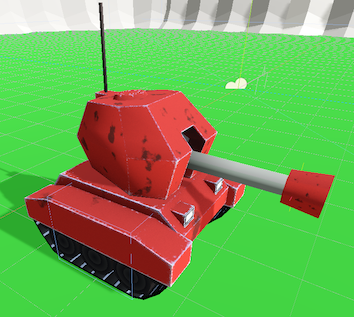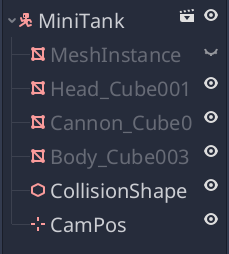# KinematicBody: Movement

## Problem

You need a player-controlled 3D kinematic body.

## Solution

For this recipe, we’ll be using this adorable tank model:You can grab this model on Itch.io: https://gtibo.itch.io/mini-tank or use any other model you’d like. We won’t be doing anything that’s tank-specific here.

We can add the model to the scene, but we’ll need a couple of additional nodes:For the collision shape, we’re just going to use a `BoxShape` aligned and sized with the tank’s treads. `CamPos` is a `Position3D` we’ll use to place our following camera. It’s placed behind and above the tank, angled down.

We’ve also rotated the individual `MeshInstance` nodes `180` degrees around the Y axis. This is because they were modeled facing towards +Z, but -Z is the forward direction in Godot, and we don’t want our tank to look like it’s backwards.

Before we add a script, open the “Project Settings” and add the following inputs on the “Input Map” tab:

Input ActionKey
forwardW
backS
rightD
leftA

Now let’s add a script, starting with the required variables:

``````extends KinematicBody

export var gravity = Vector3.DOWN * 10
export var speed = 4
export var rot_speed = 0.85

var velocity = Vector3.ZERO
``````

`speed` is the tank’s movement speed (forward and back), while `rot_speed` defines how fast it can turn.

Using the `KinematicBody.move_and_slide()` method makes our movement code quite simple:

``````func _physics_process(delta):
velocity += gravity * delta
get_input(delta)
velocity = move_and_slide(velocity, Vector3.UP)
``````

With this code, we add the downward acceleration of gravity to the current velocity, get the user’s input (more about that below), and call `move_and_slide()`. We pass the `velocity` and a direction vector of `(0, 1)` for the `up_direction` parameter.

Next we need to define `get_input()`, where we’ll process and apply the input actions:

``````func get_input(delta):
var vy = velocity.y
velocity = Vector3.ZERO
if Input.is_action_pressed("forward"):
velocity += -transform.basis.z * speed
if Input.is_action_pressed("back"):
velocity += transform.basis.z * speed
if Input.is_action_pressed("right"):
rotate_y(-rot_speed * delta)
if Input.is_action_pressed("left"):
rotate_y(rot_speed * delta)
velocity.y = vy
``````

Let’s examine this more closely. Player input should affect horizontal movement: forward/back along the ground, and rotation around the tank’s center. Movement in the Y direction should only be affected by gravity, which means we don’t want to set it to `0` every frame. This is why we’re using the `vy` variable to temporarily hold that value while we assign a new velocity vector for the horizontal movement, then add it back in at the end.

For the forward and back movement, we’re using `transform.basis.z` so that we’ll move in our body’s local forward direction.

Here’s the tank in action. We’ve made a test scene with a `StaticBody` plane for the ground and an `InterpolatedCamera` with its Target set to the tank’s `CamPos`.

## Wrapping up

This is the basis of movement for any kind of kinematic character. From here you can add jumping, shooting, AI behavior, etc. See the related recipes for examples that build on this recipe.